• 了解自动编码器作用 说明自动编码器的结构 应用 使用自动编码器对Mnist手写数字进行数据降噪处理 5.2.1 自动编码器什么用 自编码器的应用主要有两个方面 数据去噪 进行可视化而降维 自编码器可以...
学习目标

目标
了解自动编码器作用
说明自动编码器的结构

应用
使用自动编码器对Mnist手写数字进行数据降噪处理

5.2.1 自动编码器什么用

自编码器的应用主要有两个方面

数据去噪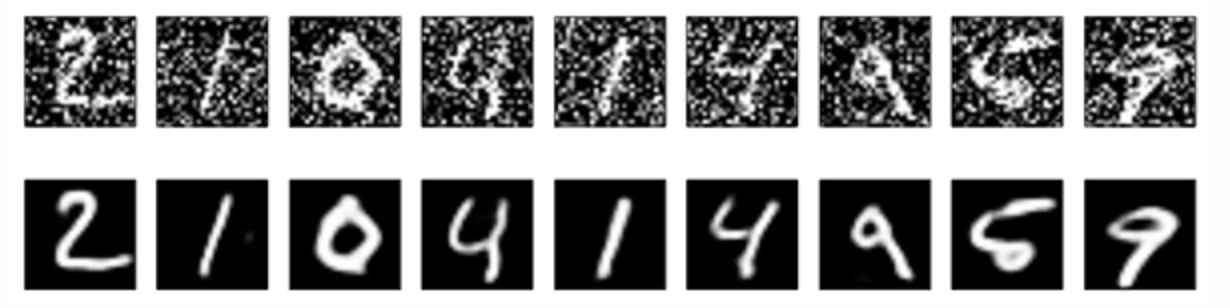进行可视化而降维
自编码器可以学习到比PCA等技术更好的数据投影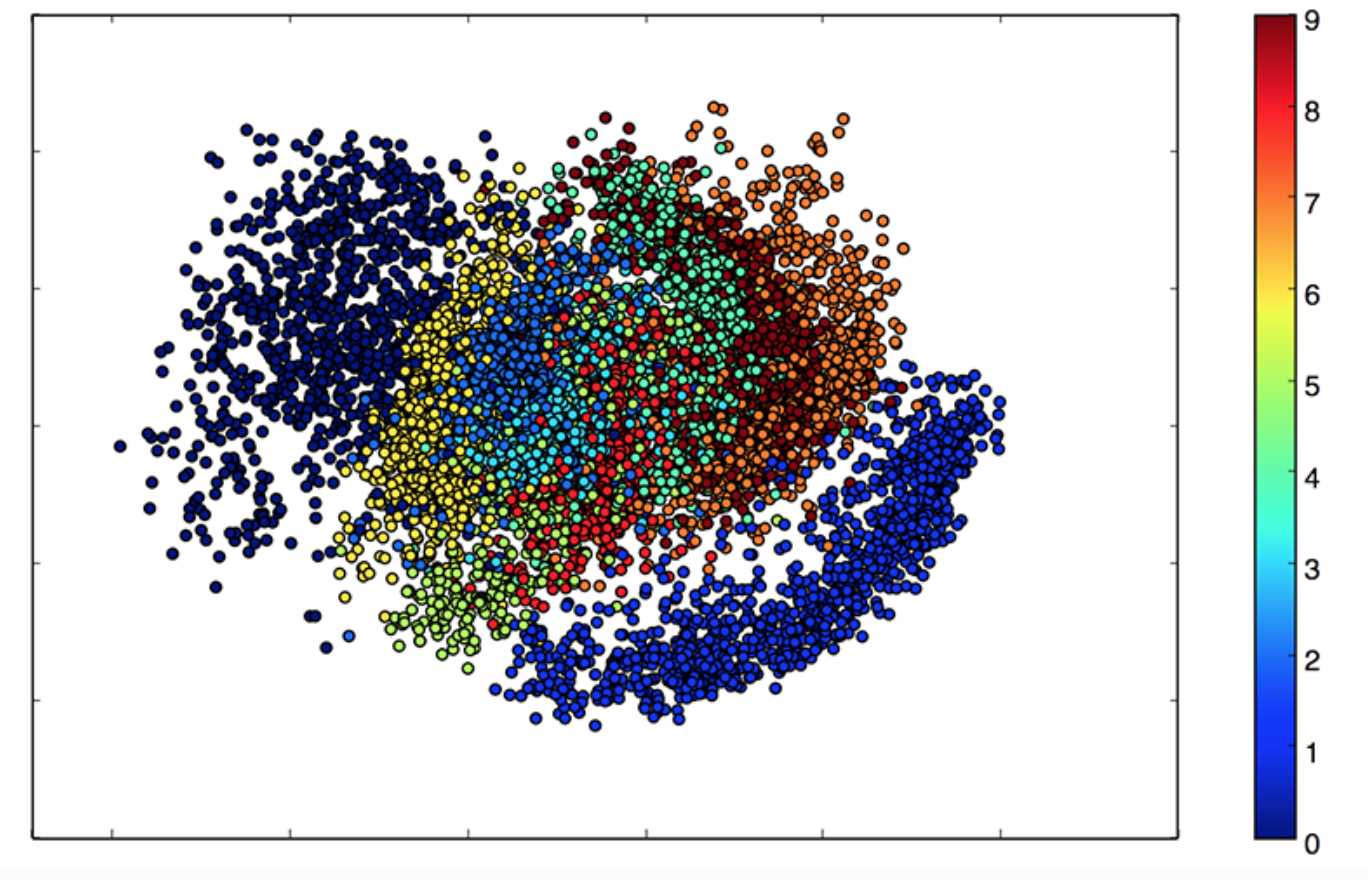5.2.1 什么是自动编码器(Autoencoder)

5.2.1.1 定义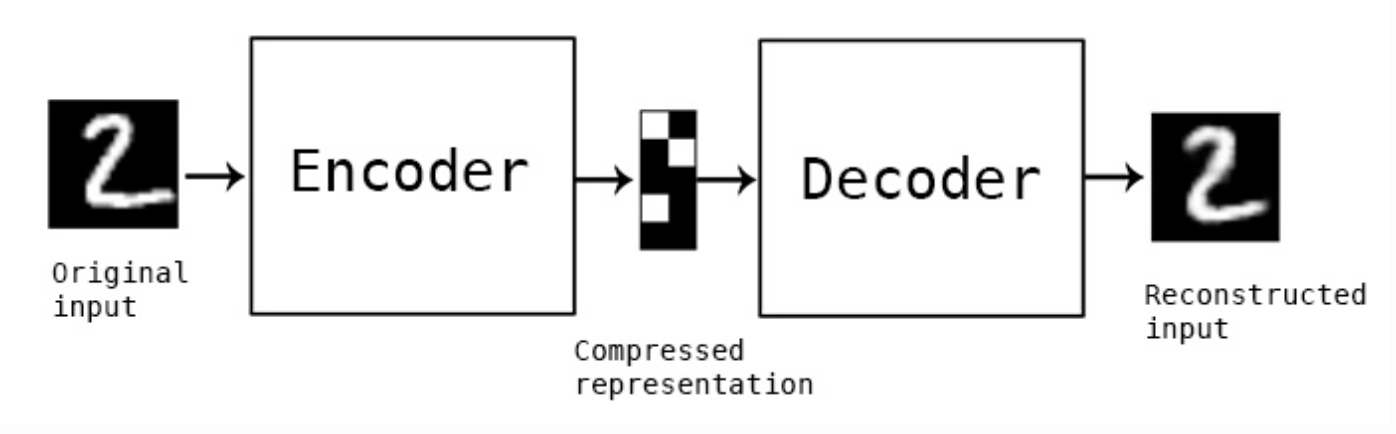自动编码器是一种数据的压缩算法，一种使用神经网络学习数据值编码的无监督方式。

5.2.1.2 原理作用案例

搭建一个自动编码器需要完成下面三样工作：

搭建编码器
搭建解码器
设定一个损失函数，用以衡量由于压缩而损失掉的信息。
编码器和解码器一般都是参数化的方程，并关于损失函数可导，通常情况是使用神经网络。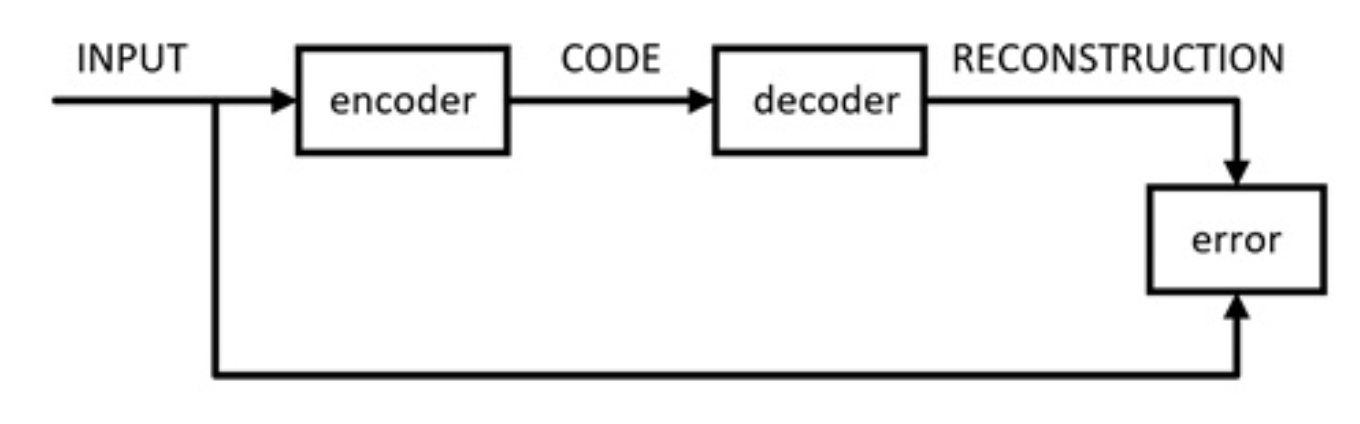5.2.1.3 类别

普通自编码器
编解码网络使用全连接层

多层自编码器
卷积自编码器
编解码器使用卷积结构

正则化自编码器
降噪自编码器

5.2.2 Keras快速搭建普通自编码器-基于Mnist手写数字

5.2.2.1 自编码器效果

迭代50次效果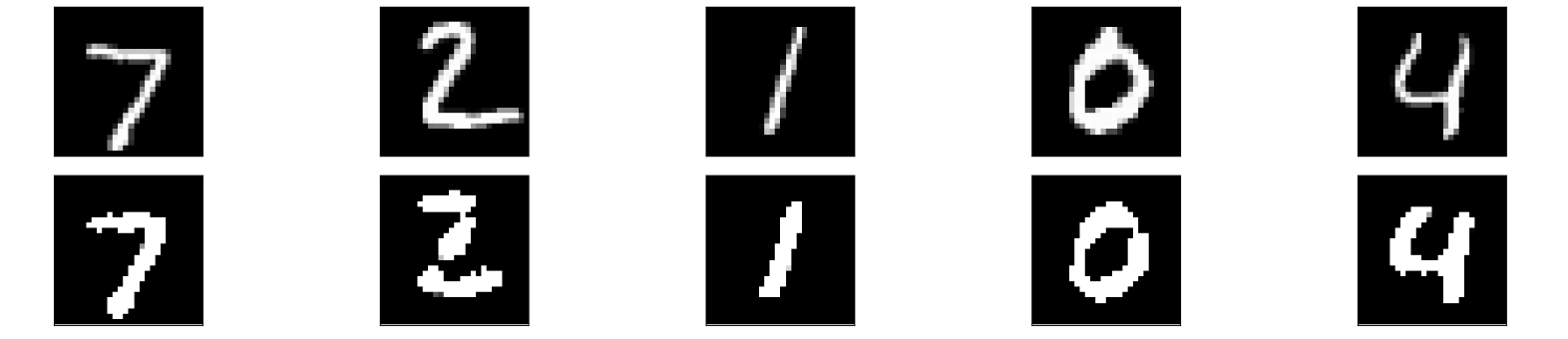Train on 60000 samples, validate on 10000 samples
Epoch 1/50
256/60000 [..............................] - ETA: 44s - loss: 0.6957
1280/60000 [..............................] - ETA: 11s - loss: 0.6867
2560/60000 [>.............................] - ETA: 6s - loss: 0.6699
3584/60000 [>.............................] - ETA: 5s - loss: 0.6493
...
...
...
55808/60000 [==========================>...] - ETA: 0s - loss: 0.0925
57088/60000 [===========================>..] - ETA: 0s - loss: 0.0925
58112/60000 [============================>.] - ETA: 0s - loss: 0.0925
59392/60000 [============================>.] - ETA: 0s - loss: 0.0925
60000/60000 [==============================] - 3s 47us/step - loss: 0.0925 - val_loss: 0.0914


5.2.2.2 流程

初始化自编码器结构
训练自编码器
获取数据
模型输入输出训练

显示自编码前后效果对比
5.2.2.3 代码编写

导入所需包

from keras.layers import Input, Dense
from keras.models import Model
from keras.datasets import mnist
import numpy as np
import matplotlib.pyplot as plt


1、初始化自编码器结构
定义编码器：输出32个神经元，使用relu激活函数，（32这个值可以自己制定）

定义解码器：输出784个神经元，使用sigmoid函数，（784这个值是输出与原图片大小一致）

损失：

每个像素值的交叉熵损失（输出为sigmoid值(0,1)，输入图片要进行归一化(0,1)）
class AutoEncoder(object):
"""自动编码器
"""
def __init__(self):

self.encoding_dim = 32
self.decoding_dim = 784

self.model = self.auto_encoder_model()

def auto_encoder_model(self):
"""
初始化自动编码器模型
将编码器和解码器放在一起作为一个模型
:return: auto_encoder
"""
input_img = Input(shape=(784,))

encoder = Dense(self.encoding_dim, activation='relu')(input_img)

decoder = Dense(self.decoding_dim, activation='sigmoid')(encoder)

auto_encoder = Model(inputs=input_img, outputs=decoder)

auto_encoder.compile(optimizer='adam', loss='binary_crossentropy')

return auto_encoder


2、训练流程
读取Mnist数据，并进行归一化处理以及形状修改
模型进行fit训练
指定迭代次数
指定每批次数据大小
是否打乱数据
验证集合

def train(self):
"""
训练自编码器
:param model: 编码器结构
:return:
"""
(x_train, _), (x_test, _) = mnist.load_data()

# 进行归一化
x_train = x_train.astype('float32') / 255.
x_test = x_test.astype('float32') / 255.

# 进行形状改变
x_train = np.reshape(x_train, (len(x_train), np.prod(x_train.shape[1:])))
x_test = np.reshape(x_test, (len(x_test), np.prod(x_test.shape[1:])))

print(x_train.shape)
print(x_test.shape)

# 训练
self.model.fit(x_train, x_train,
epochs=5,
batch_size=256,
shuffle=True,
validation_data=(x_test, x_test))


3、显示模型生成的图片与原始图片对比
导入matplotlib包

    def display(self):
"""
显示前后效果对比
:return:
"""
(x_train, _), (x_test, _) = mnist.load_data()

x_test = np.reshape(x_test, (len(x_test), np.prod(x_test.shape[1:])))

decoded_imgs = self.model.predict(x_test)

plt.figure(figsize=(20, 4))
# 显示5张结果
n = 5
for i in range(n):
# 显示编码前结果
ax = plt.subplot(2, n, i + 1)
plt.imshow(x_test[i].reshape(28, 28))
plt.gray()
ax.get_xaxis().set_visible(False)
ax.get_yaxis().set_visible(False)

# 显示编解码后结果
ax = plt.subplot(2, n, i + n + 1)
plt.imshow(decoded_imgs[i].reshape(28, 28))
plt.gray()
ax.get_xaxis().set_visible(False)
ax.get_yaxis().set_visible(False)
plt.show()


5.2.3 基于Mnist手写数字-深度自编码器

将多个自编码进行重叠
input_img = Input(shape=(784,))
encoded = Dense(128, activation='relu')(input_img)
encoded = Dense(64, activation='relu')(encoded)
encoded = Dense(32, activation='relu')(encoded)

decoded = Dense(64, activation='relu')(encoded)
decoded = Dense(128, activation='relu')(decoded)
decoded = Dense(784, activation='sigmoid')(decoded)

auto_encoder = Model(input=input_img, output=decoded)
auto_encoder.compile(optimizer='adam', loss='binary_crossentropy')


我们可以替换原来的编码器进行测试

59392/60000 [============================>.] - ETA: 0s - loss: 0.0860


最后的损失会较之前同样的epoch迭代好一些

5.2.4 基于Mnist手写数字-卷积自编码器

卷积编解码结构设计
编码器
Conv2D(32, (3, 3), activation='relu', padding='same')
MaxPooling2D((2, 2), padding='same')
Conv2D(32, (3, 3), activation='relu', padding='same')
MaxPooling2D((2, 2), padding='same')
输出大小为:Tensor("max_pooling2d_2/MaxPool:0", shape=(?, 7, 7, 32), dtype=float32)

解码器:反卷积过程
Conv2D(32, (3, 3), activation='relu', padding='same')
UpSampling2D((2, 2))
Conv2D(32, (3, 3), activation='relu', padding='same')
UpSampling2D((2, 2))
Conv2D(1, (3, 3), activation='sigmoid', padding='same')
输出大小：Tensor("conv2d_5/Sigmoid:0", shape=(?, 28, 28, 1), dtype=float32)

input_img = Input(shape=(28, 28, 1))

x = Conv2D(32, (3, 3), activation='relu', padding='same')(input_img)
x = MaxPooling2D((2, 2), padding='same')(x)
x = Conv2D(32, (3, 3), activation='relu', padding='same')(x)
encoded = MaxPooling2D((2, 2), padding='same')(x)
print(encoded)

x = Conv2D(32, (3, 3), activation='relu', padding='same')(encoded)
x = UpSampling2D((2, 2))(x)
x = Conv2D(32, (3, 3), activation='relu', padding='same')(x)
x = UpSampling2D((2, 2))(x)
decoded = Conv2D(1, (3, 3), activation='sigmoid', padding='same')(x)
print(decoded)

auto_encoder = Model(input_img, decoded)
auto_encoder.compile(optimizer='adam', loss='binary_crossentropy')


由于修改了模型的输入输出数据形状，所以在训练的地方同样也需要修改（显示的时候数据输入也要修改）

x_train = np.reshape(x_train, (len(x_train), 28, 28, 1))
x_test = np.reshape(x_test, (len(x_test), 28, 28, 1))


5.2.4 基于Mnist手写数字-降噪自编码器

降噪自编码器效果过程
对原始数据添加噪音
随机加上正态分布的噪音
x_train + np.random.normal(loc=0.0, scale=1.0, size=x_train.shape)

# 添加噪音
x_train_noisy = x_train + np.random.normal(loc=0.0, scale=3.0, size=x_train.shape)
x_test_noisy = x_test + np.random.normal(loc=0.0, scale=3.0, size=x_test.shape)

# 重新进行限制每个像素值的大小在0~1之间
x_train_noisy = np.clip(x_train_noisy, 0., 1.)
x_test_noisy = np.clip(x_test_noisy, 0., 1.)


在进行显示的时候也要进行修改

# 获取数据改变形状，增加噪点数据
(x_train, _), (x_test, _) = mnist.load_data()
x_test = np.reshape(x_test, (len(x_test), 28, 28, 1))
x_test_noisy = x_test + np.random.normal(loc=3.0, scale=10.0, size=x_test.shape)

# 预测结果
decoded_imgs = self.model.predict(x_test_noisy)

# 修改需要显示的图片变量
plt.imshow(x_test_noisy[i].reshape(28, 28))


5.2.5 总结

掌握自动编码器的结构
掌握正则化自动编码器结构作用

展开全文•MATLAB
• 主要介绍了keras自动编码器实现系列之卷积自动编码器操作，具有很好的参考价值，希望对大家有所帮助。一起跟随小编过来看看吧
• 在实际的操作中，也经常使用卷积自动编码器去解决图像编码问题，而且非常有效。下面通过**keras**完成简单的卷积自动编码。 编码器有堆叠的卷积层和池化层(max pooling用于空间降采样)组成。 对应的解码器由卷积层和...
图片的自动编码很容易就想到用卷积神经网络做为编码-解码器。在实际的操作中，也经常使用卷积自动编码器去解决图像编码问题，而且非常有效。下面通过**keras**完成简单的卷积自动编码。 编码器有堆叠的卷积层和池化层(max pooling用于空间降采样)组成。 对应的解码器由卷积层和上采样层组成。补充知识：keras搬砖系列-单层卷积自编码器考试成绩出来了，竟然有一门出奇的差，只是有点意外。觉得应该不错的，竟然考差了，它估计写了个随机数吧。头文件导入数据这里的X_train和X_test的维度分别为(60000L,784L),(10000L,784L)这里进行了归一化，将所有的数值除上255.设定编码的维数与输入数据的维数encoding_dim = 32input_img = Input(shape=(784,))构建模型模型编译autoencoder.compile(optimizer='adadelta',loss='binary_crossentropy')模型训练autoencoder.fit(X_train,X_train,epochs=50,batch_size=256,shuffle=True,validation_data=(X_test,X_test))预测encoded_imgs = encoder.predict(X_test)decoded_imgs = deconder.predict(encoded_imgs)数据可视化完成代码以上这篇keras自动编码器实现系列之卷积自动编码器操作就是小编分享给大家的全部内容了，希望能给大家一个参考，也希望大家多多支持服务器之家。原文链接：https://blog.csdn.net/xuyang508/article/details/74276181
展开全文• 量子自动编码器：经典量子（变分）自动编码器的实现
• 自动编码器，主要作用： 数据去噪， 可视化降维度， 生成数据。 模型结构： 缺点：在inference时只能通过输入一个输入得到一个隐含变量Z，然后在通过Decoder生成，无法任意去构造隐含变量。 1.2 VAE
文章目录1 VAEs1.1 AE: AutoEncoder1.2 VAE: Variational AutoEncoder1.3 CVAE: Conditional Variational AutoencoderReferences：
1 VAEs
1.1 AE: AutoEncoder
自动编码器，主要作用：

数据去噪，
可视化降维度，
生成数据。

模型结构：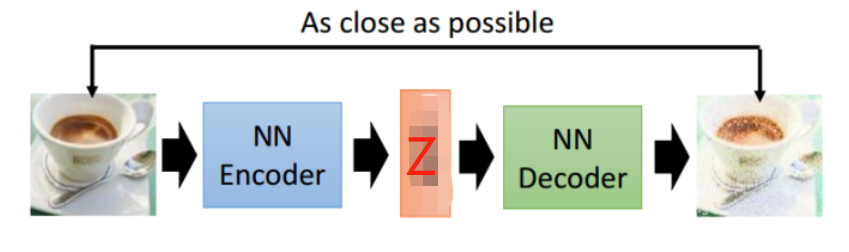缺点：在inference时只能通过输入一个输入得到一个隐含变量Z，然后在通过Decoder生成，无法任意去构造隐含变量。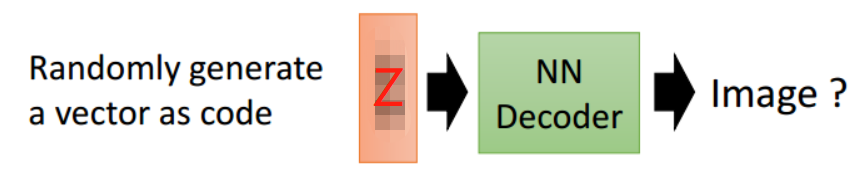1.2 VAE: Variational AutoEncoder
变分自编码器（VAE）是一个生成模型，将生成一个隐空间（latent space），从中可以对点进行采样。这些点中的任何一个都可以解码为看起来合理的target。
AE存在的问题就由VAE来解决，只需要在编码过程给它增加一些限制，迫使其生成的隐含向量能够粗略的遵循一个标准正态分布， encoder在隐空间中产生概率分布，这就是其与一般的自动编码器最大的不同，因此在Inference时，我们只需要给它一个标准正态分布的随机隐含向量，这样通过解码器就能够生成我们想要的target。
实际情况中，需要在模型的准确率上与隐含向量服从标准正态分布之间做一个权衡。

模型准确率：input和output之间求loss;

两种分布的相似程度: 使用KL divergence来表示隐含向量与标准正态分布之间差异的loss。

KL divergence 的公式：$D K L(P \| Q)=\int_{-\infty}^{\infty} p(x) \log \frac{p(x)}{q(x)} d x$，这里变分编码器使用了一个技巧“重新参数化”来解决KL divergence的计算问题。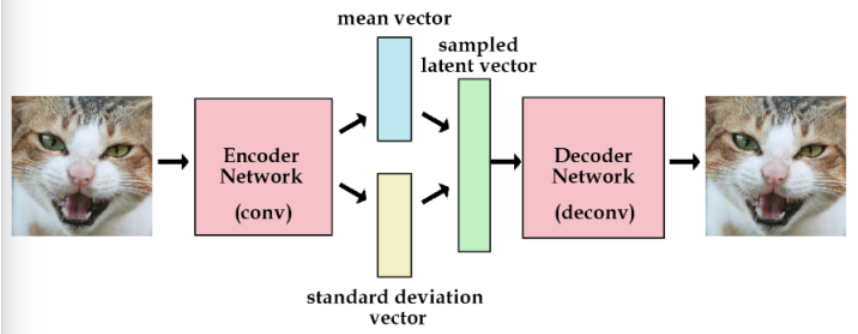这时不再是每次产生一个隐含向量，而是生成两个向量，一个表示均值，一个表示标准差，然后通过这两个统计量来合成隐含向量，这也非常简单，用一个标准正态分布先乘上标准差再加上均值就行了，这里我们默认编码之后的隐含向量是服从一个正态分布的。这个时候我们是想让均值尽可能接近0，标准差尽可能接近1。
从$N(u,\sigma^2)$采样隐含变量Z等效于，采样$\epsilon\sim N(0,1)$，然后计算$Z = u+ \sigma \cdot \epsilon$。
上述VAE中，网络中的输入和输出之间存在一个随机变量。由于无法通过随机变量反向传播梯度，这带来了一个明显的问题，即无法训练编码器。为了解决该问题，以不同的方式来表示VAE，以使隐分布的参数从随机变量的参数中被分解出来，从而可以通过隐分布的参数进行反向传播。（说白了就是采样这个过程是随机的，不能进行反向传播，所以就想办法改变VAE的表现形式使它可以被训练），具体地说，当协方差矩阵Σ是对角矩阵时，$N(u, \Sigma)=u+\Sigma N(0,1)$。重要的一点是，可以使用反向传播对VAE进行端到端训练。但是，由于仍然存在随机性因素，因此训练过程称为随机梯度变化贝叶斯（stochastic gradient variational Bayes，SGVB），而不是被称为随机梯度下降。
缺点：VAE创建了可以重现其输入的自编码器，以及可以生成合理的target的解码器，但是解码器无法按需生成特定想要的target。
对比：在传统的自编码器中，编码器从数据中获取一个样本，并返回隐空间中的单个点，然后将其传递到解码器中。在变分自编码器中，编码器会在隐空间中产生概率分布！（注意这个区别）。它输出的隐分布是与隐空间维数相同的高斯分布。编码器产生这些高斯分布的参数（均值，方差）。
1.3 CVAE: Conditional Variational Autoencoder
VAE的缺陷产生了条件变分自编码器（CVAE），它的编码器和解码器都有额外的输入。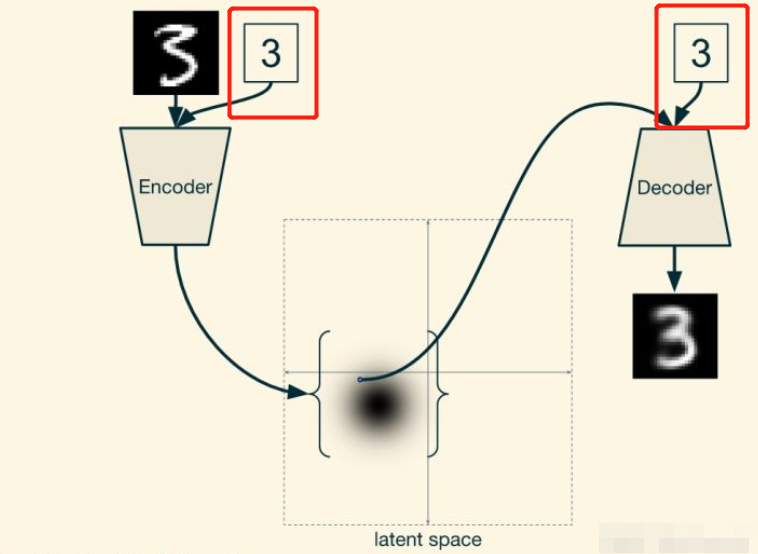在训练时，数字（标签信息）被提供给编码器和解码器。在这种情况下，它将表示为一个one-hot向量。要生成特定数字的图像，只需将该数字与从标准正态分布中采样的隐空间中的随机点一起馈入解码器即可。即使输入相同的点以产生两个不同的数字，该过程也将正确运行，因为系统不再依赖于隐空间来编码要处理的数字（标签信息）。相反，潜在空间对其他信息进行编码，例如笔划宽度或数字被写入的角度（此处我的理解是：隐空间原来编码所有输入信息，输出完全依赖于隐空间提供的采样点信息，现在在解码器处提供了标签信息，那么隐空间就编码其他信息了，相当于把标签信息从隐空间分离表征（disentangled）了）。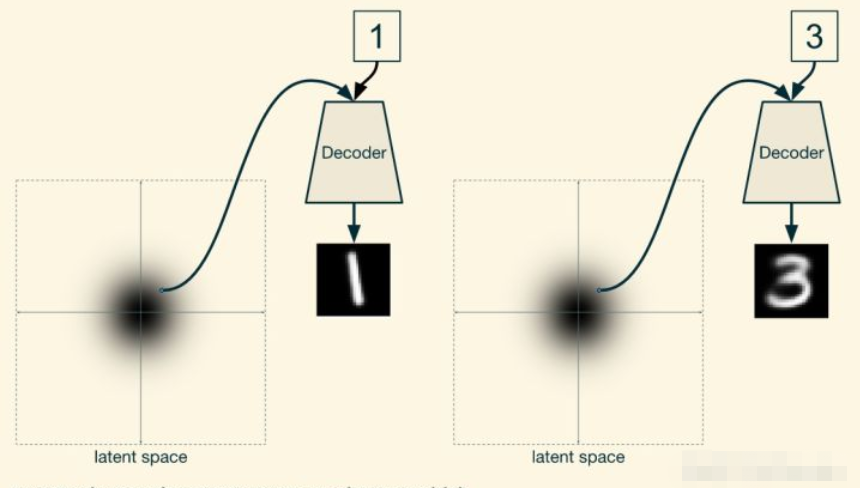References：
https://zhuanlan.zhihu.com/p/27549418
https://zhuanlan.zhihu.com/p/88750084
https://zhuanlan.zhihu.com/p/34998569
欢迎进群交流~展开全文• NNSAE：非负稀疏自动编码器（NNSAE）。 自动编码器神经网络中非负和稀疏编码的有效在线学习方案
• 2.7　实现一个自动编码器对于自动编码器，使用不同的网络架构，如图2.13 所示。在前几层中，减少了隐层神经元的数量。在中间，又开始增加隐层神经元的数量，直到隐层神经元的数量与输入变量的数量相同。中间的隐层...
2.7　实现一个自动编码器对于自动编码器，使用不同的网络架构，如图2.13 所示。在前几层中，减少了隐层神经元的数量。在中间，又开始增加隐层神经元的数量，直到隐层神经元的数量与输入变量的数量相同。中间的隐层可以看作输入的编码变体，其输出决定了编码变体的质量。在下一个方案中，将在Keras 中实现一个自动编码器，将街景门牌号(SVHN)基准库从32×32 图像解码为32 个浮点数。可以通过解码成32×32 图像并比较图像来确定编码器的质量。如何去做…1)用下面的代码导入必要的函数库：2)加载数据集并提取所需要的数据：3)预处理数据：4)接下来，定义自动编码器的网络架构：5)现在，开始训练自动编码器：6)看看自动编码如何在训练集上执行：7)绘制一些原始图像及其解码版本，如图2.14 所示。在第3 章　卷积神经网络　中，将向读者展示如何为SVHN 数据集实现一个卷积自动编码器。喜欢的朋友可以添加我们的微信账号：51CTO读书频道二维码51CTO读书会第9群:808517103【责任编辑：book TEL：(010)68476606】点赞 0
展开全文• 3D自动编码器 该项目旨在开发具有3D卷积层的自动编码器。 图书馆Tensorflow 2Python
• VAE（Variational Auto Encodes）自动编码器是无监督学习的一种。深度学习
•研究论文
•研究论文
• 自动编码器原理： 由编码器和解码器组成，编码器和解码器通常是神经网络模型。 输入的数据经过神经网络降维到一个编码，再通过一个神经网络去解码得到一个与输入原始数据一模一样的生成数据，然后通过比较这两个数据...机器学习
• TensorFlow 2.0中的自动编码器 以下自动编码器的实现； 香草自动编码器（AE） 去噪自动编码器 备用自动编码器（进行中） 压缩式自动编码器（进行中） 可变自动编码器（VAE） 条件变分自动编码器（CVAE） 节拍...
• 在实际的操作中，也经常使用卷积自动编码器去解决图像编码问题，而且非常有效。 下面通过**keras**完成简单的卷积自动编码。 编码器有堆叠的卷积层和池化层(max pooling用于空间降采样)组成。对应的解码器由卷积层和...卷积神经网络 keras
• 自动编码器(autoencoder) 是神经网络的一种，该网络可以看作由两部分组成：一个编码器函数h = f(x) 和一个生成重构的解码器r = g(h)。传统上，自动编码器被用于降维或特征学习自编码器原理示意图编码器：将原始高维...
• 学习使用Python中的Keras包，利用自动编码器Autoencoder，分析手写字体数据。首先是准备工作，程序如下：## 加载包%matplotlib inline%config InlineBackend.figure_format='jpeg'from IPython.display import Image...
•HTML
• 来源 | 数据派 THU概述自动编码器是什么？自动编码器是如何工作的？本文将回答这些问题。我们将通过一个案例——如何提高模糊图像的分辨率，来探讨自动编码器的概念。简介你还记得胶卷相机的...
•研究论文...